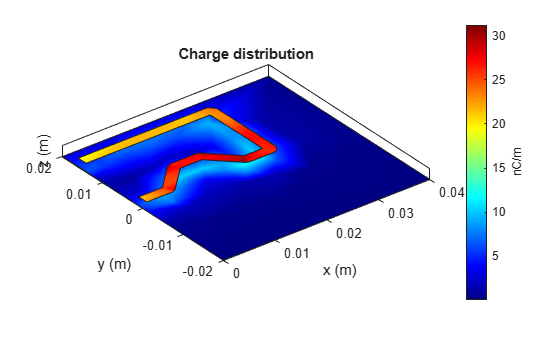# Create and Analyze PCB Interconnects using Custom Traces

This example shows how to use the custom traces `traceLine` and `tracePoint `in RF PCB Toolbox and create PCB traces with different shapes and orientations. The `traceLine` uses the lengths and angles properties to create a trace and the `tracePoint` uses points to create the trace. For both the traces, corner property can be set to `Sharp`, `Miter,` and `Smooth` to create specific corners at all the bends.

### Line Trace

Use the `traceLine` object to create a line trace. Observe that the traceLine has Length, Angle, Width, and Corner as properties. The line trace is a combination of all the rectangles which are created based on the `Length, Angle`, and the `Corner` property is used to specify the type of corner at each bend. You can specify the corners as sharp, miter, or smooth . By default the `Corner` property is set to `Sharp`.

`trace = traceLine`
```trace = traceLine with properties: Name: 'mytraceLine' StartPoint: [0 0] Length: [0.0200 0.0200 0.0200 0.0150] Width: 0.0050 Angle: [90 0 -90 45] Corner: "Sharp" ```

Visualize the traceLine using the `show` function.

```figure; show(trace);```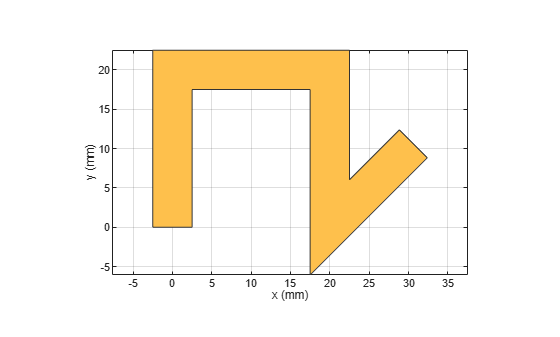Change the `Length` and `Angle` property on the traceLine and set the `Corner` to `smooth`.

```trace.Length = [10 5*sqrt(2) 10 5*sqrt(2) 10]*1e-3; trace.Angle = [0 45 0 -45 0]; trace.Width = 3e-3; trace.Corner = "smooth"; figure; show(trace);```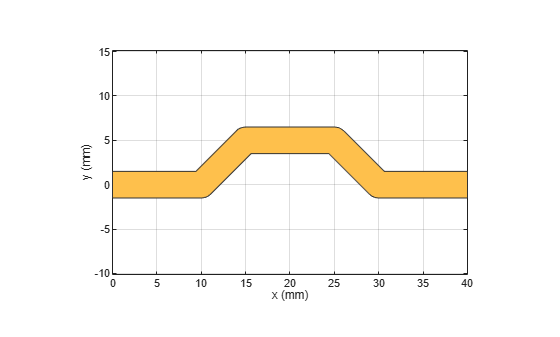The output shows that the trace is created using the angle specified for each corresponding length input. The corners are specified as smooth and hence it creates curved edges.

### Extend the Line Trace

Add a 15 mm line perpendicular to the earlier trace. So add 15 mm to the `Length` property and 90 deg to the `Angle` property of the trace and set the corner to `Miter.`

```trace.Length = [10 5*sqrt(2) 10 5*sqrt(2) 10 15]*1e-3; trace.Angle = [0 45 0 -45 0 90]; trace.Width = 3e-3; trace.Corner = "Miter"; figure; show(trace);```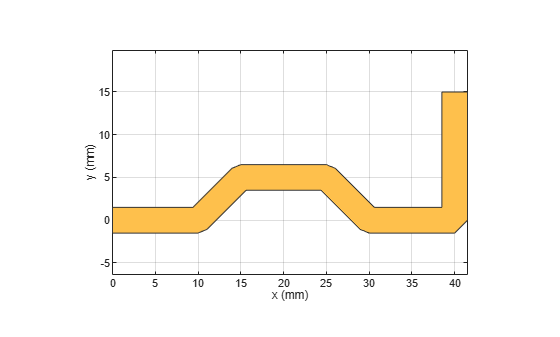The output shows that the trace is created using the angle specified for each corresponding length input. The corners is specified as Miter and hence it creates mitered edges. Observe that the ends of the trace are at [0,0] and [40,15] mm where the feed points are assigned in the next section.

### Create PCB Component

Use the `pcbComponent` to create the PCB stack for the shape. For creating a PCB stack, use the trace created as the top layer. The middle layer is a dielectric and the bottom layer is ground plane. Use the `dielectric` object to create the FR4 dielectric. Use `traceRectangular` object to create a rectangular ground plane. Assign the `trace`, dielectric(`d`), and `groundplane` to the `Layers` property on the `pcbComponent`. Assign the `FeedLocations` at the ends of the trace and visualize it.

```pcb = pcbComponent; d = dielectric('FR4'); d.Thickness = pcb.BoardThickness; groundplane = traceRectangular('Length', 60e-3,'Width',30e-3,'Center',[60e-3/2,0]); pcb.Layers = {trace,d,groundplane}; pcb.BoardShape = groundplane; pcb.FeedDiameter = trace.Width/2; pcb.FeedLocations = [0,0,1,3;40e-3,15e-3,1,3]; figure; show(pcb);```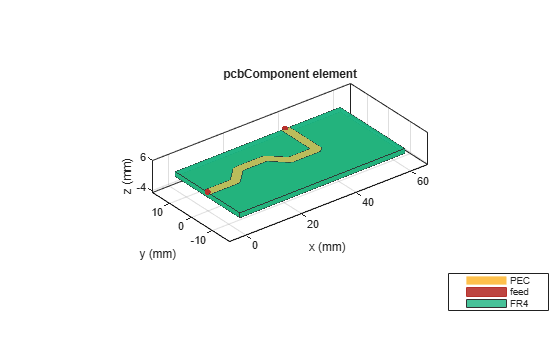Use `sparameters` to calculate the s-parameters of the trace and plot it using `rfplot` function.

```spar = sparameters(pcb,linspace(1e9,5e9,51)); figure; rfplot(spar);```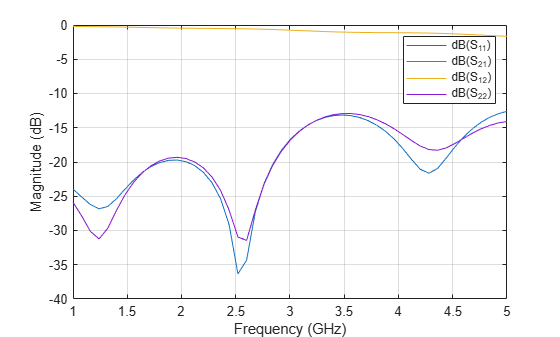### Point Trace

Use the tracePoint object to create a Point trace. Observe that the `tracePoint` has `TracePoints`, `Width,` and `Corner` as properties. Based on the Points, the tracePoint creates the trace and the Corner property is used to specify the type of corner at each bend i.e. you can specify either sharp, miter or smooth. Change the `TracePoints` and set the corner poperty to `Miter`

```trace = tracePoint; trace.TracePoints = [0 0;5 0;10 5;15 5;20 0;25 0]*1e-3; trace.Corner = "Miter"; figure; show(trace);```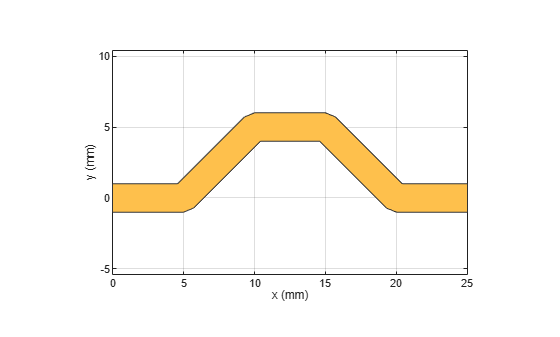### Extend the trace

Add an addional L-Shaped trace to the earlier trace so that the feed points are aligned to the same edge. Add two points in the `TracePoints` property as shown below. Set the `Corner` to `Miter`.

```trace.TracePoints = [0 0;5 0;10 5;15 5;20 0;25 0;25 15;0 15]*1e-3; trace.Corner = "Miter"; figure; show(trace);```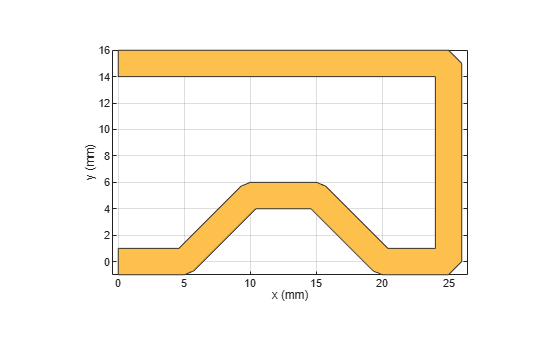### Create PCB Component

Use the `pcbComponent` to create the PCB stack for the shape. For creating a PCB stack, use the trace created as a top layer. The middle layer is a dielectric and the bottom layer is ground plane. Use the `dielectric` object to create the FR4 dielectric. Use `traceRectangular` object to create a rectangular ground plane. Assign the `trace`, dielectric(d) and `groundplane` to the `Layers` property on the `pcbComponent`. Assign the `FeedLocations` at the ends of the trace and visualize it.

```pcb = pcbComponent; d = dielectric('FR4'); d.Thickness = pcb.BoardThickness; groundplane = traceRectangular('Length', 40e-3,'Width',40e-3,'Center',[40e-3/2,0]); pcb.Layers = {trace,d,groundplane}; pcb.FeedLocations = [0,0,1,3;0e-3,15e-3,1,3]; pcb.BoardShape = groundplane; pcb.FeedDiameter = trace.Width/2; figure; show(pcb);```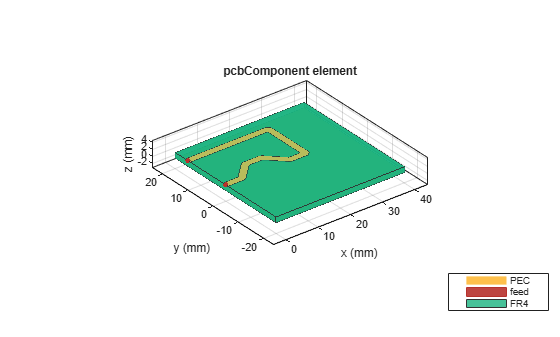Use `current` function to plot the current distribution on the trace

```figure; current(pcb,1e9);```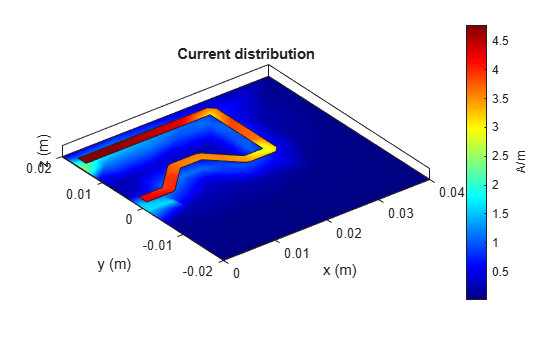Use `charge` function to plot the charge on the trace

```figure; charge(pcb,1e9);```